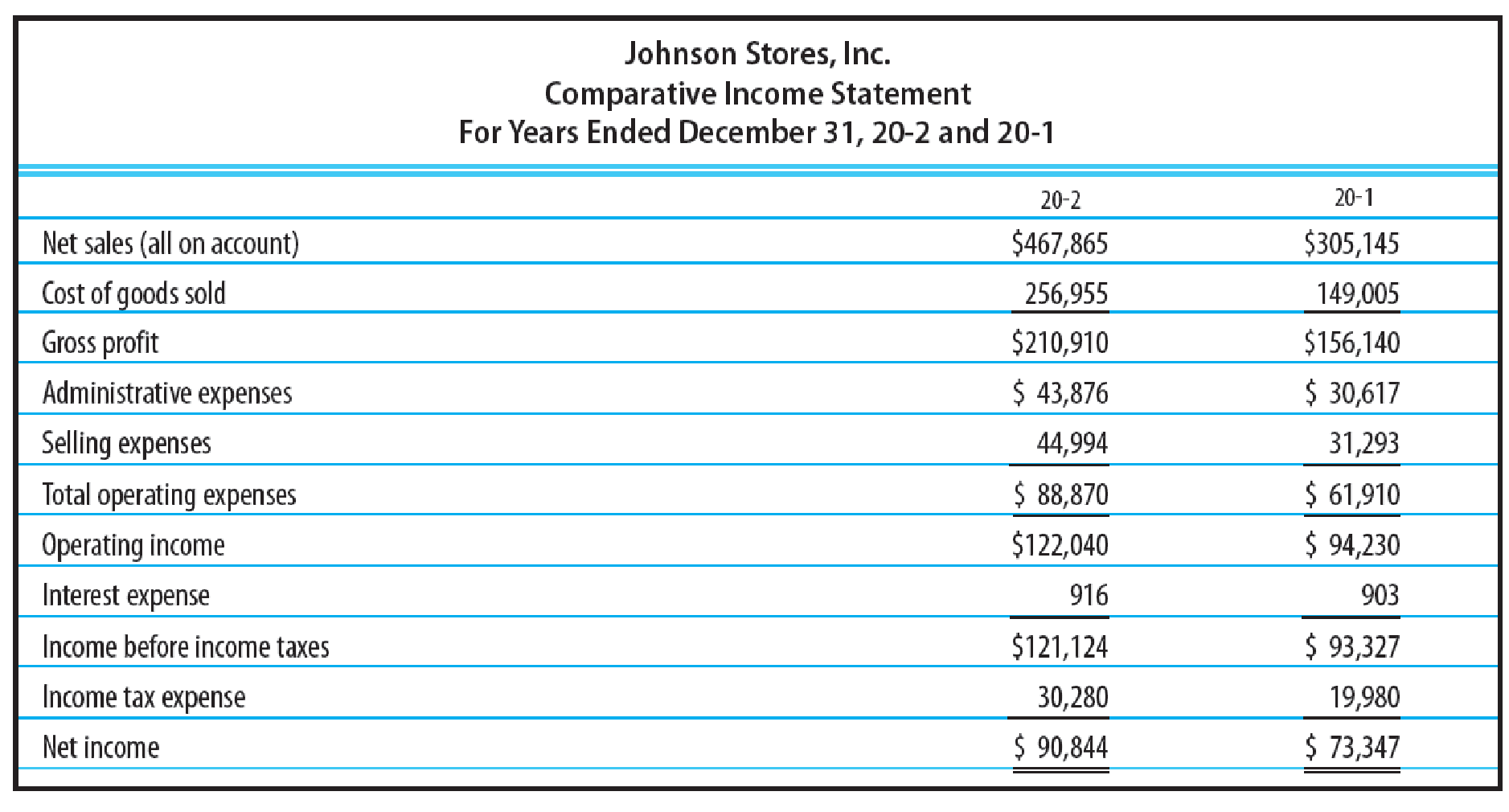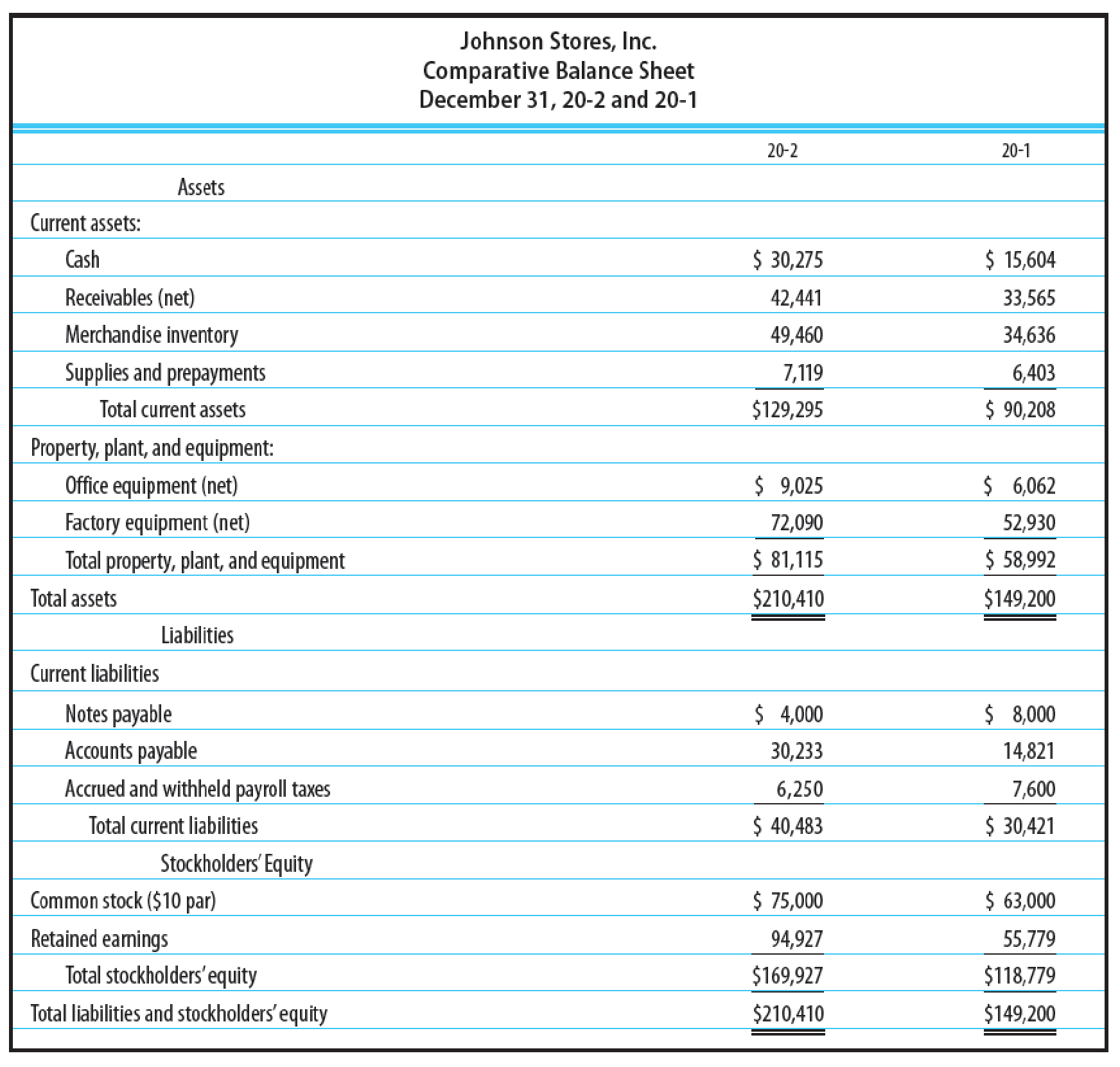# HORIZONTAL ANALY SIS OF COMPARATIVE FINANCIAL STATEMENTS Amounts from the comparative income statement and balance sheet of Johnson Stores, Inc. For the last two years are as follows: REQUIRED Prepare a horizontal analysis of the statements. Add columns to show the amount of increase (decrease) and the percentage change. Round percentages to one decimal place. VERTI CAL ANALY SIS OF COMPARATIVE FINANCIAL STATEMENTS Refer to the financial statements in Problem 24-8B. REQUIRED Prepare a vertical analysis of the income statement and balance sheet. Show each item on the income statement as a percentage of the net sales for each year. On the balance sheet, show each asset item as a percentage of the total assets and each liability and equity item as a percentage of the total liabilities and stockholders’ equity. Round percentages to one decimal place.### College Accounting, Chapters 1-27 ...

22nd Edition
James A. Heintz + 1 other
Publisher: Cengage Learning
ISBN: 9781305666160

#### Solutions

Chapter
Section### College Accounting, Chapters 1-27 ...

22nd Edition
James A. Heintz + 1 other
Publisher: Cengage Learning
ISBN: 9781305666160
Chapter 24, Problem 9SPB
Textbook Problem
10 views

## HORIZONTAL ANALY SIS OF COMPARATIVE FINANCIAL STATEMENTS Amounts from the comparative income statement and balance sheet of Johnson Stores, Inc. For the last two years are as follows:REQUIREDPrepare a horizontal analysis of the statements. Add columns to show the amount of increase (decrease) and the percentage change. Round percentages to one decimal place.VERTI CAL ANALY SIS OF COMPARATIVE FINANCIAL STATEMENTS Refer to the financial statements in Problem 24-8B.REQUIREDPrepare a vertical analysis of the income statement and balance sheet. Show each item on the income statement as a percentage of the net sales for each year. On the balance sheet, show each asset item as a percentage of the total assets and each liability and equity item as a percentage of the total liabilities and stockholders’ equity. Round percentages to one decimal place.

To determine

Prepare vertical analysis for income statement and balance sheet.

### Explanation of Solution

Vertical analysis: The comparison of an item of a financial statement against the total amount of the same financial statement, to determine the relation between the two items, is referred to as vertical analysis. This analysis is also known as common-size analysis. The vertical analysis percentage is calculated by using the formula:

Verticalanalysispercentage=FinancialstatementitemBaseamount×100

Prepare vertical analysis for income statement as shown below:

 Incorporation JS Comparative Income Statement For Years Ended December 31, 20-2 and 20-1 Particulars 20-2 20-1 Amount Percent Amount Percent Net sales (all on account) $467,865 100$305,145 100 Cost of goods sold 256,955 54.9 149,005 48.8 Gross profit $210,910 45.1$156,140 51.2 Administrative expenses $43,876 9.4$30,617 10 Selling expenses 44,994 9.6 31,293 10.3 Total operating expenses $88,870 19$61,910 20.3 Operating income $122,040 26.1$94,230 30.9 Interest expense 916 0.2 903 0.3 Income before income taxes $121,124 25.9$93,327 30.6 Income tax expense 30,280 6.5 19,980 6.5 Net income $90,844 19.4$73,347 24

Table (1)

Prepare vertical analysis for balance sheet statement as show below:

 Johnson Stores, Inc. Comparative Balance Sheet December 31, 20-2 and 20-1 Particulars 20-2 20-1 Amount Percent Amount Percent Assets Current assets: Cash \$30,275 14

### Still sussing out bartleby?

Check out a sample textbook solution.

See a sample solution

#### The Solution to Your Study Problems

Bartleby provides explanations to thousands of textbook problems written by our experts, many with advanced degrees!

Get Started

Find more solutions based on key concepts
Why is productivity important?

Essentials of Economics (MindTap Course List)

Explain the following statement: Although the balance sheet can be thought of as a snapshot of a firms financia...

Fundamentals of Financial Management, Concise Edition (with Thomson ONE - Business School Edition, 1 term (6 months) Printed Access Card) (MindTap Course List)

Explain how the CPI is computed.

Economics (MindTap Course List)